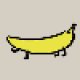Jump to content
• Advertisement# kotoko

Member

6

116 Neutral

## About kotoko

• Rank
Newbie
1. Hello all, I'm after a way to check for frame buffer support to automatically adjust what I'm using. How do I check for this in Java? Example: Start my java program that will render water. I want to know if frame buffers are supported so I choose how I'm going to represent the water: a simple plane or a filter with ripples, reflection and whatnot. I'm using jMonkeyEngine3 that uses lwjgl to get to the opengl.
2. Hello all, I'm having trouble choosing the normal vector to order my triangle vertexes. So I have the 3 points that define a plane, I did the subtraction - got 2 vectors, did cross product got the normal. My problem now is I don't understand how to choose between the ordering I get and the opposite to make sure I define a surface that is from above, just like a terrain. Some psedo-code to explain better: [source lang="java"]private boolean isCounterClock(Vector3f p1, Vector3f p2, Vector3f p3) { Vector3f a1 = p1.subtract(p2); Vector3f a2 = p3.subtract(p2); Vector3f normal = a2.crossLocal(a1).normalizeLocal(); // the normal vector for the downwards face must always have negative y if (normal.y < = 0f) { return true; } else { return false; } } [/source] What am I doing wrong?
3. I see. I actually have yaw-pitch-roll, just didn't know it was easier. I'll use Pi for location and your system for the vectors. Is this written in any kind of tutorial/book/crash course?
4. Thank you so much for your answer! I have a few questions though. Sorry if they are very basic. The data I have acess to is (using your notation) Pi, Pi+1 and Phi or the Roll angle. I know that f = (Pi+1)-Pi For u, the idea was to rotate the unitary y vector according to Phi. But I don't remember how to apply a rotation to a vector. Finally, for s, I was planning to calculate the normal of f and u. How do I rotate?
5. Hello everyone, I have the points for a vehicle trajectory in 3D space now I want to make a triangle strip that shows the vehicle trajectory. Like in this image I have the line, now I need the strip. My main question is how to calculate the x(width) and z(depth) since depending on slope it's not as simple as adding half the width of the segment. Since I have two points in the line and the slope, I should be able to calculate what is the point at D distance from the line points. I just can't put my finger on the math. Can anyone help me out?
6. Hello all, I'm implementing b-spline curves and there is one last point I'm not understanding. What doest the parameter (t or u in most explanations) range correspond to in visual terms? I understand how to calculate the values to compute C(t) = ? Bi , p (t )Pi but I don't understand how to vary t (or u). What I want is to know what are the points between P1 and P2, how do I do that by varing the t? This is probably obvious question, sorry
• Advertisement
×

## Important Information

By using GameDev.net, you agree to our community Guidelines, Terms of Use, and Privacy Policy.

GameDev.net is your game development community. Create an account for your GameDev Portfolio and participate in the largest developer community in the games industry.

Sign me up!